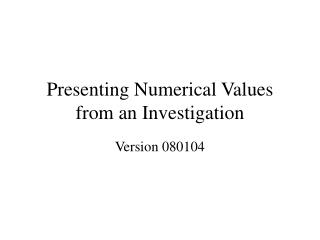DownloadDownload PresentationPresenting Numerical Values from an Investigation

# Presenting Numerical Values from an Investigation

Download Presentation## Presenting Numerical Values from an Investigation

- - - - - - - - - - - - - - - - - - - - - - - - - - - E N D - - - - - - - - - - - - - - - - - - - - - - - - - - -
##### Presentation Transcript

1. Presenting Numerical Values from an Investigation Version 080104

2. Outline • Uncertainty • Propagation of Uncertainty • Least Squares Fit to Lines

3. Online References • www.av8n.com/physics/uncertainty.htm • mathworld.wolfram.com/LeastSquaresFitting.html • www.physics.csulb.edu/151lab/exp4/lsf.html

4. Which is the best measurement?Which measurement is consistent with the previously published result? • 6.63 E-34 Js • 6.7 E-34 Js • 6.623 E-34 Js

5. Which is the best measurement?Which measurement is consistent with the previously published result? • 6.613 ±0.003 E-34 Js • 6.7 ±0.2 E-34 Js • 6.622±0.001 E-34 Js

6. UNCERTAINTY

7. Uncertainty CJ’s bad karma Best Power Supply money can buy 30 yr old piece of precision equipment Best measurements that can be performed Voltage stabilization circuit built by Floyd Vibrations from Levi Center construction

8. Cumulative Set of Measurementsby Lab Unit #1 # of Occurances

9. Cumulative Set of Measurementsby Lab Unit #2 # of Occurances should report center and width of distribution

10. 45±1 45±2 may look like numbers, but they’re not.It’s a way to specify a probability distribution

11. Relative Uncertainty An experiment measures the value of the fundamental charge to be: (1.84 ± 0.09) x 10-19 Coulombs or 1.14 ± 0.06 e

12. SignificantFigures

13. Sig Figs are for Losers “Non-experts tend to talk about ‘significant digits’, but this approach is heavily flawed. Professionals general prefer to speak in terms of quantifying the uncertainty.”

14. Why Sig Fig approach is bad An experiment measures the value of the fundamental charge to be: 1.1 e 1.149 1.050 10% rel unc. is implied Sig Fig approach: 1) misrepresents the true uncertainty (actually the uncertainty is not specified) 2) throws away information 3) can produce erroneous results (see linear fits) Physicists present a ‘reasonable number’ of digits in published work, but many more digits in calculations.

15. Example Calculational tools for an investigation return the result: 1.64578359 ± 0.05385672 meters Q: In a professional paper this should be presented as (?): 1.6458 ± 0.0539 meters 1.646 ± 0.054 meters 1.65 ± 0.05 meters 1.6 ± 0.1 meters

16. Notation • 1.646 ± 0.054 meters • 1.646 (54) meters • 1.646 ± 3% meters preferred for written work preferred for conversation

17. Types of Uncertainty • Statistical • Systematic

18. What is the “Correct” Answer? • “In classroom settings, people often get the idea that the goal is to report an uncertainty that reflects the difference between the measured value and the ‘correct’ value.” • “That idea certainly doesn’t work in real life – if you knew the ‘correct’ value you wouldn’t need to make measurements.”

19. PROPAGATION OF UNCERTAINTY

20. ?

21. LEAST SQUARES FITTING Figures and equations from: mathworld.wolfram.com/LeastSquaresFitting.html

22. y = a0 + a1 x + a2 x2 + … The Excel “trendline” function isn’t good enough. One must always present uncertainties to be a member of the physics community. Use “linest” to get the parameters and their corresponding uncertainities. Use “trendline” to draw the graph.

23. Two possible ways to select the best line:

24. Minimize square-deviation wrt choice of coefficients assume linear fn

25. intercept a = slope b =

26. Using the Excel function LINEST Slope = INDEX(LINEST(y-values,x-values,TRUE,TRUE),1,1) Uncertainty in Slope = INDEX(LINEST(y-values,x-values,TRUE,TRUE),2,1) Intercept = INDEX(LINEST(y-values,x-values,TRUE,TRUE),1,2) Uncertainty in Intercept = INDEX(LINEST(y-values,x-values,TRUE,TRUE),2,2)